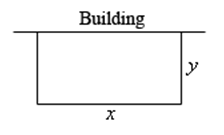# What is Optimization ?

Mathematical optimization is the selection of the best element based on a particular criterion from a set of available alternatives.

In simple cases, a specific optimization problem involves minimizing or maximizing or real function systematically by choosing input values within an allotted set and finding the function’s value. It applies a large area of mathematics for generalizing theory of optimization. Optimization involves determining “best available” values of the particular objective function in a defined domain along with a variety of different types of objective functions.

Now, let’s have a look at optimization problems. Here, you need to look for the greatest or the smallest value that can be taken by a function. The constraint will be normal that can be described by an equation.

Also, to remember that the constraint is the quantity that has to be true irrespective of the solution. You will be looking at one quantity that is clear and has a constant value in every problem. Once you identify the quantity to be optimized, it’s not difficult to proceed.

## Optimization Example

Let us see the solved example on optimization concept for better understanding.

Example: A field has to be enclosed with a fence. You have 500 feet of material, and the building is on one side of the field and not required to be fenced. Calculate the dimensions of the field that will close the largest region.

Solution:

In these problems, we have two functions. The first is the function that you will optimize, and second is the constraint. You can sketch the situation that can help you to arrive at the equation.Here, we want to maximize the area of the field, and we have 500 ft material for fencing. Therefore, the area (i.e. area of a rectangle) will be the function that has to be optimized and the constraint is the amount of fencing. Hence, the two equations are:

Maximize: A = xy

Constraint: 500 = x + 2y

If you solve the constraint for one of the variables, you can substitute it into the area and then get a function of a single variable.

Let’s solve the constraint for x.

x = 500 – 2y

Now, substitute it in the area function that provides a function of y.

A(y) = (500 – 2y)y = 500y – 2y2

Now, you need to find the largest value on the interval [0, 250]. Note that the interval corresponds to considering y = 250, i.e., no width and two sides and both must be 250 ft to utilize the whole 500 ft. Also, the endpoints of the interval won’t mean anything if we want to enclose some region both would give zero area. However, they give us a set of limits on y and the Extreme Value Theorem, which says that we will have a maximum value of the area between two endpoints.

To know more about optimization and related articles regarding optimization techniques, applications of derivatives and calculus, you can visit us at www.byjus.com